Home

# ON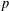$p$ -PARTS OF CONJUGACY CLASS SIZES OF FINITE GROUPS

## Abstract

Let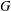$G$ be a finite group. Let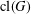$\operatorname{cl}(G)$ be the set of conjugacy classes of$G$ and let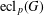$\operatorname{ecl}_{p}(G)$ be the largest integer such that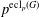$p^{\operatorname{ecl}_{p}(G)}$ divides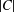$|C|$ for some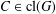$C\in \operatorname{cl}(G)$ . We prove the following results. If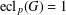$\operatorname{ecl}_{p}(G)=1$ , then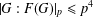$|G:F(G)|_{p}\leq p^{4}$ if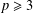$p\geq 3$ . Moreover, if$G$ is solvable, then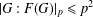$|G:F(G)|_{p}\leq p^{2}$ .

## Footnotes

Hide All

The project was supported by NSFC (Nos. 11671063 and 11471054), the Natural Science Foundation Project of CSTC (cstc2016jcyjA0065) and the NSF of Jiangsu Province (No. BK20161265). The first author was also supported by a grant from the Simons Foundation (No. 499532).

## References

Hide All
MathJax
MathJax is a JavaScript display engine for mathematics. For more information see http://www.mathjax.org.

# ON$p$ -PARTS OF CONJUGACY CLASS SIZES OF FINITE GROUPS

## Metrics

### Full text viewsFull text views reflects the number of PDF downloads, PDFs sent to Google Drive, Dropbox and Kindle and HTML full text views.

Total number of HTML views: 0
Total number of PDF views: 0 *Loading metrics...

### Abstract viewsAbstract views reflect the number of visits to the article landing page.

Total abstract views: 0 *Loading metrics...

* Views captured on Cambridge Core between <date>. This data will be updated every 24 hours.

Usage data cannot currently be displayed.